## conference papersJOURNAL OFAPPLIEDCRYSTALLOGRAPHY
ISSN: 1600-5767
Volume 40| Part s1| April 2007| Pages s88-s92

## Simplified tube form factor for analysis of small-angle scattering data from carbon nanotube filled systems

aAir Force Research Laboratory, Materials and Manufacturing Directorate, WPAFB, OH 45433-7750, USA, bDepartment of Chemical and Materials Engineering, University of Cincinnati, Cincinnati, OH 45221-0012, USA, and cUniversity of Dayton Research Institute, 300 College Park, Dayton, OH 45469-0168, USA
*Correspondence e-mail: dale.schaefer@uc.edu

(Received 16 August 2006; accepted 25 January 2007; online 17 February 2007)

This work presents an analysis method for small-angle scattering data utilizing a simplified tube (hollow cylinder) form factor. The simplified form factor captures the rod-like character of a tube at long length scales (one-dimensional), the sheet-like character of the tube wall at intermediate length scales (two-dimensional), and the surface characteristics of a tube at small length scales while suppressing the deep minima seen in the exact form factor. Ultra-small-angle X-ray scattering data from composites made with multi-walled carbon nanotubes and a bismaleimide resin are analyzed using the simplified form factor and compared with scanning electron micrographs. Although a hollow core is not evident via microscopy, a solid rod form factor does not fit the data. However, a tube form factor does fit the data and generates reasonable geometric parameters. At higher concentrations, evidence for aggregation is seen in the data. Aggregation is accommodated by including a fractal structure factor within the simplified approach, allowing facile analysis of data from aggregated (poorly dispersed) fillers.

### 1. Introduction

It is widely appreciated that aggregation is detrimental to the mechanical properties of composites reinforced with carbon nanotubes. Therefore it is paramount to develop techniques to assess quantitatively both the morphology and degree of aggregation of low-dimensional reinforcing fillers. Because it is a non-destructive, in situ, volume-sensitive probe, small-angle scattering is an obvious candidate to provide the required morphological data. By combining measurements from light scattering, ultra-small-angle scattering, and small-angle scattering, one can obtain morphological information for complex systems over length scales from a few Ångströms to a few hundred micrometres (Bauer et al., 2006; Hough et al., 2006; Wang et al., 2004; Yurekli et al., 2004; Zhou et al., 2004; Schaefer, Brown et al., 2003; Schaefer, Zhao et al., 2003). Analysis of scattering profiles, however, is susceptible to misinterpretation due to over zealous analysis of the characteristically featureless profiles. Nevertheless, reasonable conclusions can be drawn from the absolute intensity of the scattering over a broad range of length scales (Brown et al., 2005; Schaefer, Brown et al., 2003). In this work, we develop simplified form factors to facilitate such analysis.

Using a simplified tube form factor (STFF), we analyze ultra-small-angle X-ray scattering (USAXS) data obtained for multi-walled carbon nanotubes (MWCNTs) and bismaleimide (BMI) composites. The accuracy of the analysis is verified by comparison with images obtained via scanning electron microscopy (SEM). We first derive a STFF to describe hollow cylinders (tubes) and compare the STFF with the known analytic tube form factor. We show the experimental scattering intensity from USAXS is inconsistent with scattering from a solid cylinder by comparing fits generated with a simplified rod (solid cylinder) form factor to fits generated by the STFF. By considering the tube wall, we take into account the increased surface area and decreased overall volume that a tube has compared with a rod thus improving the fit.

We enhance the utility of the STFF by considering long-range fractal correlations thus generating a fractal-tube form factor. The benefit to having such a tool is we can determine the degree of aggregation as well as distinguish whether the nanotubes remain hollow or become solid (filled) after processing.

### 2. Experimental

#### 2.1. Sample preparation

Preparation of samples for USAXS was based upon procedures described by Wilkinson (1991) for toughening BMI resin. The desired quantity of MWCNTs [catalytically grown MWCNTs (>90% pure); MER Corporation] were functionalized as previously reported (Baek et al., 2004) and mixed with the appropriate quantity of Matrimid 5292B [o,o′-diallyl bisphenol A; Huntsman Advanced Materials (Europe)] using an Ultra-Turrax T25 homogenizer (300 rev s−1, 423 K, 3 h). The MWCNTs/Matrimid 5292B was then sonicated in an ethylene glycol bath (80 kHz/150 W, 373 K, 4 h). After sonication, the mixture was heated in a silicone oil bath (423 K) and a stoichiometric amount (Wilkinson, 1991; Yee & Pearson, 1983) of Matrimid 5292A [1,1′-(methylenedi-p-phenylene) bismaleimide; Huntsman Advanced Materials (Europe)] was added to the MWCNT/Matrimid 5292B mixture. Fig. 1shows the chemical structures of composite constituents.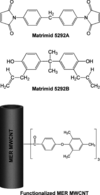Figure 1 Components used in the sample preparation for USAXS. The functionalization shown for the MWCNT occurs on the available carbon sites at a ratio of 3:100 (arylcarbonyl group:carbon site).

The filled resin was then placed in heated silicon molds (423 K) designed to make 1 mm-thick composite coupons. The molds were covered with a flat silicon caul sheet and bagged for autoclave curing (Brown et al., 2000). The coupons were cured under vacuum in a compression mold at a pressure of 690 kPa and temperatures of 453 K (2 h) and 472 K (4 h), with a 5 K min−1 temperature ramp. The composites remained in the autoclave until the system returned to ambient temperature.

#### 2.2. Ultra-small-angle X-ray scattering

USAXS provides information about real-space structures ranging in size from 1 nm to 1 µm. Structural information is gained by measuring the scattering intensity, I, as a function of the modulus of the scattering vector, q = (4π/λ)sin(θ/2), where λ is the wavelength of the radiation in the medium, and θ is the scattering angle. The USAXS intensity I(q) is measured on an absolute scale as the differential scattering cross-section per unit sample volume per solid angle (Ω) subtended by the detector, I(q) = dΣ/(VdΩ). Note that q has the units of reciprocal length so scattering at a given q is sensitive to real space inhomogeneities on the scale q−1.

The USAXS data in this work were taken at the X-ray Operations and Research beamline 33-ID-D at the Advanced Photon Source (APS) at Argonne National Laboratory, Argonne, USA. The Bonse–Hart USAXS camera at this facility covers the regime 3 × 10−4q ≤ 0.1 Å−1. The wavelength of the incident X-rays was 1.00 Å. The data were desmeared using Indra routines provided by Jan Ilavsky at the APS.

### 3. Results and discussion

#### 3.1. Simplified tube form factor (STFF)

Previous attempts to model carbon nanotube scattering data using a simplified-rod form factor motivated the development of the STFF (Brown et al., 2005; Zhao et al., 2005). Although an analytic form factor is available for both rods and tubes, these expressions are intractable, particularly in the case of tubes. Furthermore, the oscillations in the exact form factors require averages over distributions of tube radii and/or wall thickness in order to analyze data. Such averages are computationally demanding due to the multiple nested integrations required. Such integrations virtually eliminate the possibility of iterative optimization of size distributions. These limitations motivated the need for an algebraic approximation to the exact functions.

Fig. 2compares the simplified rod and tube form factors with the analytic tube form factor. Code used to generate the analytic form factor was written at the NIST Center for Neutron Research, National Institute of Standards and Technology, Gaithersburg, USA. Fig. 2emphasizes the limitations of the simplified-rod form factor when attempting to fit tube data. The rod form factor can match the exact tube form factor at either high or low q, but not both. Furthermore, deviations occur at intermediate q due to the two-dimensional character of the tube wall. In contrast with the rod, the STFF matches the analytic counterpart, albeit the oscillations are suppressed.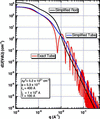Figure 2 Comparison between simplified and analytic form factors. The simplified tube fits the global shape of the exact tube form factor but suppresses the deep minima. Using the same dimensional parameters, the simplified-rod form factor (Zhao et al., 2005) over estimates the intensity at small q (because the volume is too high) and underestimates the intensity at high q (because the surface area is too low).

#### 3.2. STFF equations and fitting parameters

The strategy behind the STFF is to match the amplitude of the exact form factor in power-law scattering regimes and join the power-law regions with Guinier-like crossovers following the unified approach to data analysis (Beaucage, 1995; Beaucage & Schaefer, 1994). If L is the length of the tube, T is the wall thickness, ro is the outer radius and ri is the inner radius, the scattering intensity in four distinct regimes can be estimated under certain approximations. For example for q << 1/L, I(q) is given by the Guinier prefactor, G. Assuming L >> ro >> T: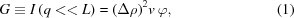where Δρ is the difference in scattering length density between the tube wall and the pure matrix material, v is the volume of a single tube (excluding the hollow region), and φ is the volume fraction occupied by the wall material.

As the q values increase to q ≃ (Rg)−1, where Rg is the radius of gyration of one tube given by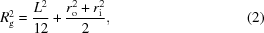the crossover is given by the Guinier approximation (Roe, 2000):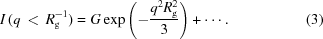At larger q, we expect the scattering to arise from the rod-like features of the tube. Therefore, in the region (Rg)−1 < q < (ro)−1, the scattering will scale like scattering from a slender rod,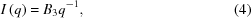where B3 is the prefactor yet to be determined, and the exponent on q is the fractal dimension of the scatterer, which is −1 for a one-dimensional object. We use the notation Bsubscript for the power-law prefactors, where the subscript identifies the level' of the unified fit (Beaucage, 1995; Beaucage & Schaefer, 1994), with the highest subscript referring to the lowest q region.

To determine B3, we make the analogy to the B value for a solid rod, which we previously determined (Zhao et al., 2005) to be: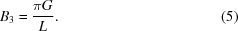As expected, B3 scales as the cross-sectional area of the tube.

In the range (ro)−1 < q < (rori)−1, the intensity is expected to follow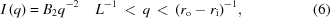where the exponent −2 is characteristic of a two-dimensional object. To determine B2, we make an analogy to scattering from a flat sheet, which we know from a previous study of the simplified disk form factor (Schaefer et al., 2005):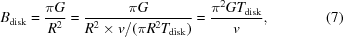where R is the disk radius and Tdisk is the disk thickness. By this analogy, B2 for a tube should be: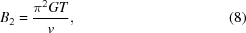where T = (rori) is the wall thickness, which is assumed to be small compared with ro. The prefactor is proportional to the wall thickness as expected, but in this case, the prefactor is not obvious.

Finally, for smooth tube walls, the high-q power-law is calculated from Porod's law: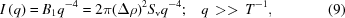where Sv is the interfacial area per unit volume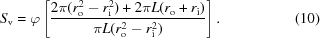Therefore, in terms of G, the equation for B1 is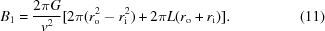The STFF utilizes Beaucage's unified approach (Beaucage, 1995; Beaucage & Schaefer, 1994) to smoothly link the Guinier and power-law regimes. The complete equations for the STFF are given by equations (12)–(16). In these equations, the Guinier crossover lengths, ro and T, in equations (14)–(15)were determined by comparison with the analytic tube form factor.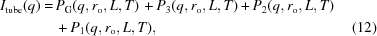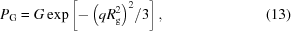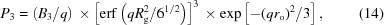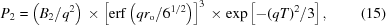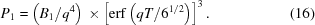#### 3.3. Long-range correlations

The STFF is adequate for rigid, isolated, monodisperse tubes. However, polydispersity in tube dimensions, tube flexibility, and/or long-range correlations cause the STFF to fit the data inadequately. Polydispersity can be handled by averaging over distribution(s) of the three lengths in the problem, although such averaging does not seem to be necessary for the problem at hand. Attributes such as flexibility and/or long-range correlations can be accounted for by multiplication of the STFF by a fractal-structure factor, such as was derived by Teixeira (1988). We know from previous work (Schaefer, Brown et al., 2003; Schaefer, Zhao et al., 2003; Brown et al., 2005) that nanotubes dispersed in a solution or a polymer matrix typically exhibit flexibility and often exist as aggregated clusters even in well dispersed' samples.

If we assume the tube is not rigid – that is, the tube exhibits behavior similar to a self-avoiding walk – the tube can be modeled as a worm-like chain. Thus, the tube is considered to be flexible on large scales and stiff on short length scales. The assumed morphology of the chain-like tube is based on fractal ordering of short tube-like segments with a persistence length Lp. These short (Lp << L), rigid segments are correlated on larger length scales with a fractal dimension, D. For example, if the resulting objects followed a self-avoiding walk, D = 5/3. Objects with branching would have a larger value of D, as would a random-walk chain. The structure factor S(q) proposed by Teixeira (1988) for fractal correlations is given by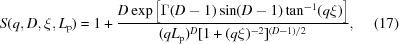where Γ(x) is the gamma function and ξ is the correlation range, which is taken to be the radius of the `cluster' resulting from the arrangement of the segments. Moreover, the utilization of this structure factor combined with STFF results in the overall fractal-tube form factor, IF-Tube, which provides a generic description the flexible tube morphology: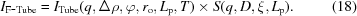This expression can also be utilized if the tubes are aggregated into clusters or if the tubes are branched. The physical meaning of D, ξ and Lp would change, but equation (18)should still be valid if branching or clustering is present.

#### 3.4. Modeling MWCNTs/BMI composite USAXS data

USAXS data reported on an absolute scale for two loadings (0.01 and 0.05 wt%) of MWCNTs in BMI resin are shown in Fig. 3(all % listed are weight %). A background scan of the carbon-free cured BMI resin was subtracted from each sample, so presumably all scattering originates from the MWCNTs dispersed throughout the matrix. Furthermore, the scattering is attributed to carbon since the intensity scales with carbon loading. To emphasize the power-law scattering at high q, a flat background was subtracted from each data set.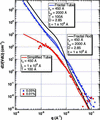Figure 3 USAXS data for MWCNTs/BMI composites compared with simplified form factors. The 0.01% data fit with the STFF, but the form factor will not fit the 0.05% data due to long-range correlations. The fractal rod and fractal-tube form factors account for the long-range correlations although the fractal rod still cannot sufficiently fit the data.

The 0.01% data fit well using the STFF without considering long-range correlations. This fact is consistent with dispersion down to the level of individual tubes. The intensity, however, is close to background at low q, so the data are insufficient within the q range where scattering from aggregated clusters would occur. Therefore we cannot unambiguously conclude aggregation is not present.

Figs. 4(a) and (b) are SEM images of the MER MWCNTs after functionalization and before mechanical mixing with the resin. Simple observation of these images allows one to estimate roughly the diameters of the MWCNTs to be ≈ 800 Å. Unfortunately, no estimations concerning wall thickness of the tubes can made from these images.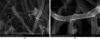Figure 4 SEM images, (a) and (b), of the MER MWCNTs functionalized similarly to previous work (Baek et al., 2004) and used in the composites. The diameters of the nanotubes after functionalization are ~800 Å, which validates the dimensions obtained from USAXS using the STFF and the fractal-tube form factor.

When the loading of MWCNTs is increased to 0.05%, aggregation must be present since no region with power-law scattering with a slope of −1 is visible at low q (Fig. 3). If we use the same 450 Å radius used when fitting the 0.01% sample, it is impossible to fit the data with the STFF since long-range correlations are not considered, and the STFF forces a slope of −1 at low q. A fractal-rod form factor (Fig. 3) also fails to fit the data since it cannot fit the data at low and high q simultaneously due to the higher surface area and lower material volume of tubes compared with rods. However, the fractal-tube form factor is designed to consider all of the geometric attributes of the tubes and is able to adequately fit the high-concentration data when aggregation is present. Since the fractal-rod form factor does not fit the 0.05% data and the fractal-tube form factor does with reasonable tube dimensions (compared with the 0.01% sample and SEM), we conclude that the tubes must be hollow even though hollow cores are not evident via SEM. We have assumed in the derivation of the STFF that the core is filled with the matrix material.

### 4. Conclusion

This work presents a simplified tube form factor that captures all of the features of the analytical tube form factor in a more user-friendly, algebraic expression. The STFF is shown to be more accurate than a simplified-rod form factor when used to analyze USAXS data from carbon-nanotube-reinforced composites. However, the STFF is inadequate when flexibility or aggregation is present in tube morphology. To account for these features, long-range fractal correlations are considered by the implementation of a long-range fractal-structure factor. The resulting fractal-tube form factor is shown to have greater utility than a fractal-rod form factor when considering tube morphologies. Whereas the fractal-rod form factor is unable to simultaneously fit scattering data from tubes at low q (because the volume of a rod is too large) and high q (because the surface area of a rod is significantly lower), the fractal-tube form factor is able to account for these features. As a result, one can determine whether the cylinders are hollow (tubes) or solid (rods) based upon which form factor fits the data more accurately.

It must be recognized that exact models do exist for calculating form factors for anisotropic objects (Kotlarchyk & Chen, 1983; Roess & Shull, 1947; Pedersen & Schurtenberger, 1996; Shull & Roess, 1947). Simplification of these existing approaches is the motivation for this work. By developing this STFF based on algebraic approximations, we are able to reduce significantly the calculation complexity while still capturing the salient morphological features of the scatterers. We show the analysis of scattering profiles that arise from tubes can be approximated using a series of relatively simple algebraic expressions combined with a unified approach to curve fitting.

### Acknowledgements

The authors thank David Anderson (University of Dayton Research Institute) and Janis Brown (Air Force Research Laboratory) for their help with sample preparation. We also thank Gary Price (University of Dayton Research Institute) for taking the SEM images. This research was partially funded by the United States Air Force Research Laboratory through the Wright Brothers Institute and contract F33615-00-D-5006. The USAXS data presented in this work were collected on the X-ray Operations and Research beamline 33-ID-D at the Advanced Photon Source, Argonne National Laboratory, with assistance in collection and reduction from Jan Ilavsky. The use of the Advanced Photon Source was supported by the U. S. Department of Energy, Office of Science, Office of Basic Energy Sciences, under Contract No. W-31-109-ENG-38.

### ReferencesJOURNAL OFAPPLIEDCRYSTALLOGRAPHY